header > paper > figures > footer

# Enhancing the Visualization of Characteristic Structures in Dynamical Systems

by H. Löffelmann and E. Gröller.

Project Duration: 1997 - 1998.

This page assembles some results (figures) of work that is part of our research topic ``Visualization of Complex Dynamical Systems''. The figures are provided in JPEG format.header < paper > figures > footerheader < paper < figures > footer

## Figures in the paper (JPEG)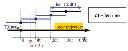Figure 1: Relation between streamlet density (no), streamlet integration length (len), and streamlet instantiation interval (dt)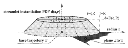Figure 2: Probability density function d for the instantiation of streamlets based on a perpendicular cross-section through the base trajectory.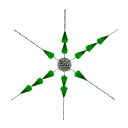Figure 3a: Visualizing the flow near a linear node repellor in 3D: eigenvectors and eigenvalues.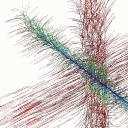Figure 3b: Visualizing the flow near a linear node repellor in 3D: characteristic trajectories plus threads of streamlets.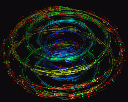Figure 4a: A thread of streamlets visualizing the flow near a torus in three-space.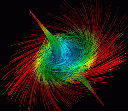Figure 4b: Flow near a 3D focus visualized using two threads of streamlets.Figure 5a: Visualizing the flow velocity near a stream line of the Roessler system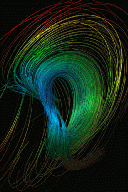Figure 5b: Visualizing the dynamics of a periodic dynamical system exhibiting a twisted torusheader < paper < figures < more images < footer

Helwig Löffelmann, last update on January 30, 1998.